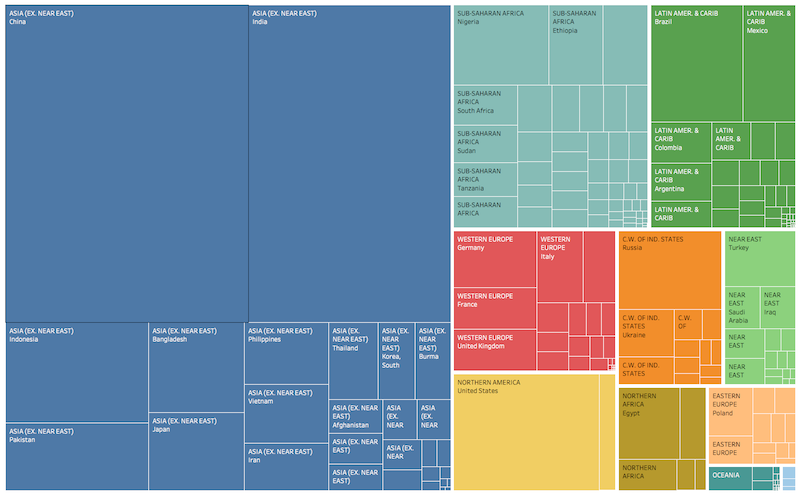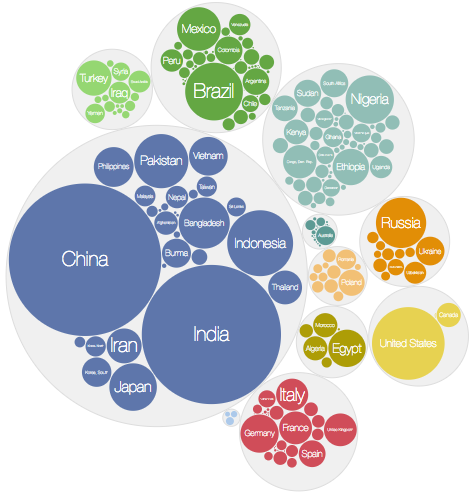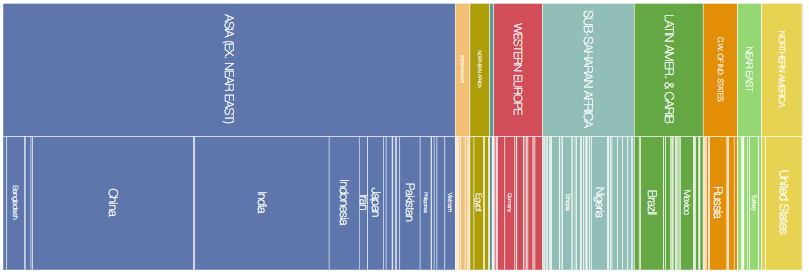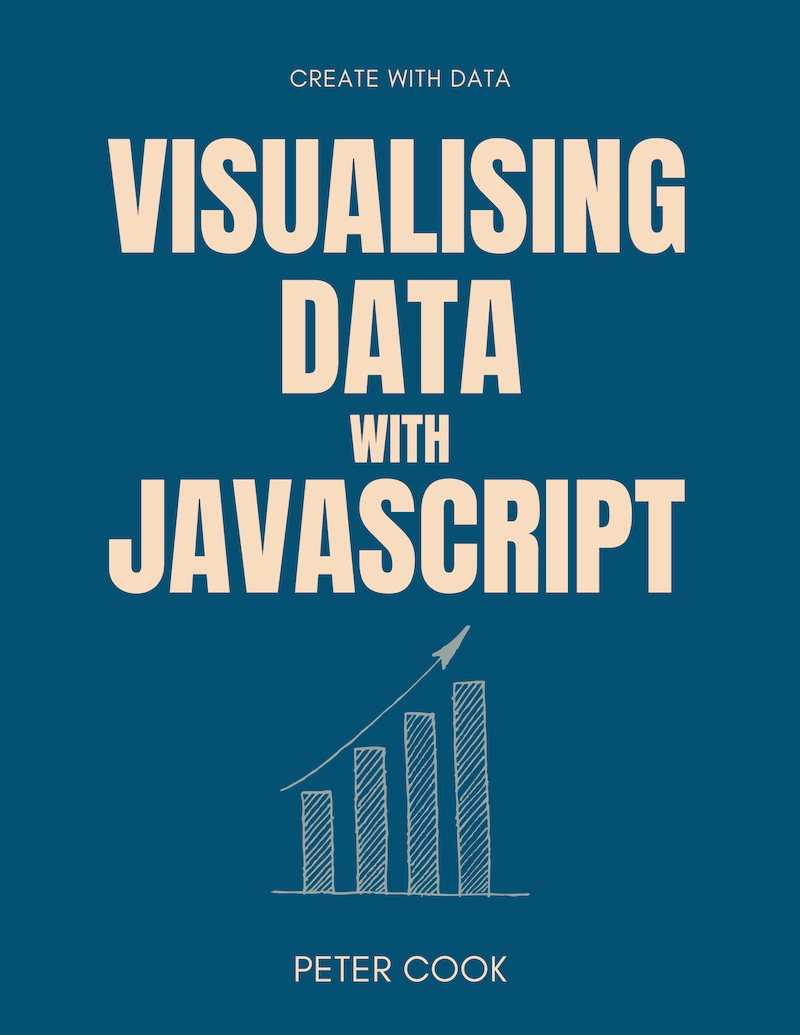D3 in Depth covers versions 6 and 7 of D3

Get book discounts and receive D3.js related news and tips.

Subscribe

# D3 Hierarchies

How to visualise hierarchical data (data in the shape of trees) using D3.js. This article shows how to create a nested (or hierarchical) data structure from an array of data. It also covers visualising hierarchies using D3, including treemaps, circle packing and sunburst charts.

A common technique when analysing or visualising data is to organise your data into groups.

For example, here's some film data:

TitleDistributorGenreWorldwide_GrossRotten_Tomatoes_Rating
Air BudWalt Disney PicturesComedy2755506145
Air Force OneSony PicturesAction31526835378
Alex & EmmaWarner Bros.Drama1535858311
AliSony PicturesDrama8438396667
All the King's MenSony PicturesDrama952145811

Let's group it according to Distributor and count the number of films in each group:

DistributorCount
Sony Pictures4
Walt Disney Pictures3
Warner Bros.3

You can also group by more than one category. For example let's group by Distributor, then group by Genre:

DistributorGenreCount
Sony PicturesAction1
Comedy1
Drama2
Comedy1
Drama2

In the world of spreadsheets, the above table is also known as a pivot table. If you're familiar with databases you can achieve similar affects using the SQL statement `GROUP BY`.

The data how has a hierarchical structure. At the top level are Distributors (Sony Pictures, Walt Disney Pictures etc.) and at the next level are Genres (Action, Adventure, Comedy etc.).

You can think of a hiearchical structure as a tree-like struture where a root item (or 'node') splits into top level groups (Distributors in our example). Each top level group splits into second level groups (Genres in our example), and so on:

The topmost item (or node) is known as the root node. The bottommost items are known as leaves or leaf nodes. In the leaf nodes of the above example, the count is displayed under the dot.

You can also aggregate (or 'rollup') the groups in different ways. For example you can sum `Worldwide_Gross`:

DistributorGenreSum of Worldwide_Gross
Sony PicturesAction315268353
Comedy22498520
Drama93905424
Comedy27555061
Drama67331612

There are several ways in which hierarchical data can be visualised including trees, treemaps, packed circles and sunbursts. Each of these can be created using D3 and we'll cover each of them later in this chapter.

First we'll look at how D3 can help us create a hierarchical data structure from flat data.

## Creating a hierarchy from an array of data

Given an array of data such as:

``let data = [  {    "Title": "Adaptation",    "Distributor": "Sony Pictures",    "Genre": "Comedy",    "Worldwide_Gross": 22498520,    "Rating": 91  },  {    "Title": "Air Bud",    "Distributor": "Walt Disney Pictures",    "Genre": "Comedy",    "Worldwide_Gross": 27555061,    "Rating": 45  },  {    "Title": "Air Force One",    "Distributor": "Sony Pictures",    "Genre": "Action",    "Worldwide_Gross": 315268353,    "Rating": 78  },  ...];``

you can use D3's `.rollup` function to group the data by any of the categorical properties.

The above array is similar to the output of d3.csv. (See requests chapter.)

The first argument of `.rollup` is the array you're wanting to group.

The next argument is a reduce function. This is a function that takes an array of values and outputs a single value. For example it might iterate through the array, summing one of the properties (such as `Worldwide_Gross`).

The remaining argument(s) are functions which specify which properties to group by.

Let's group by `Distributor` and `Genre` and sum `Worldwide_Gross` across the items in each group:

``function sumWorldwideGross(group) {  return d3.sum(group, function(d) {    return d.Worldwide_Gross;  });}let groups = d3.rollup(data,                       sumWorldwideGross,                       function(d) { return d.Distributor; },                       function(d) { return d.Genre; }                      );``

In the above example `d3.rollup` groups `data` by `Distributor` and `Genre`. Each of the groups is then passed into `sumWorldwideGross` which returns the sum of `Worldwide_Gross`.

`d3.rollup` returns a nested map object. (Maps, just like regular JavaScript objects, hold key-value pairs. You can read more about them here.)

You can inspect the map returned by `d3.rollup` using `.get`:

``// Get Sony Picturesgroups.get('Sony Pictures');  // {"Comedy" => 22498520, "Action" => 315268353, "Drama" => 93905424}// Get Drama within Sony Picturesgroups.get('Sony Pictures').get('Drama');  // 93905424``

The first `.get` returns the group associated with `Sony Pictures`. This group contains 3 items: `Comedy`, `Action` and `Drama`. (You can compare this with the table we showed previously.)

The second `.get` returns the value associated with `Drama` within the `Sony Pictures` group (93905424). This returns the value computed by the rollup function (`sumWorldwideGross`) because we're at the lowest level of the hieararchy.

## d3.hierarchy structure

D3 has a bespoke hierarchy data structure that gives you some useful features over and above the map object seen previously.

It's created by calling `d3.hierarchy` and passing in the map object generated by `d3.rollup`:

``function sumWorldwideGross(group) {  return d3.sum(group, function(d) {    return d.Worldwide_Gross;  });}let groups = d3.rollup(data,                       sumWorldwideGross,                       function(d) { return d.Distributor; },                       function(d) { return d.Genre; }                      );let root = d3.hierarchy(groups);``

You can also pass a nested object into `d3.hierarchy`. The layout examples later on use this approach.

The output of `d3.hierarchy` is a nested object that looks something like:

``{  data: [undefined, Map(3)],  children: [    {      data: ["Sony Pictures", Map(3)],      children: [...],      depth: 1,      height: 1,      parent: {...} // this item's parent node    }.    {      data: ["Walt Disney Pictures", Map(2)],      children: [...],      depth: 1,      height: 1,      parent: {...} // this item's parent node    }.    {      data: ["Warner Bros.", Map(3)],      children: [...],      depth: 1,      height: 1,      parent: {...} // this item's parent node    }  ],  depth: 0,  height: 2,  parent: null}``

It's similar in structure to the map object that's passed into `d3.hierarchy`. The difference is that it's a regular JavaScript object and has various properties and methods defined on it that provides additional functionality.

Each item (or node) in the hierarchy has properties: `data`, `children`, `depth`, `height` and `parent`.

`data` is the associated item from the map or object that's passed into `d3.hierarchy`. In this example it's a two-element array containing the group name and the group's value. For leaf nodes (the nodes at the bottom-most level), the value is the aggregated value (for example, the sum of `Worldwide_Gross`). Otherwise, it's the map representing the items in the group. Typically you won't need to access the value because the hierarchy makes this data available through its `children` and `value` properties.

`children` is an array containing the node's children. `depth` and `height` indicate the depth and height of the node within the hierarchy. (The root node has a depth of zero and leaf nodes have a height of zero.)

`parent` references the node's parent node.

The leaf nodes look something like:

``{  data: ["Comedy", 22498520],  depth: 2,  height: 0,  parent: {...} // this item's parent node}``

You can see that the `data` property contains the rolled up value. If the rolled up value is a sum or count, it can be be propagated back up the tree using the hierarchy's `.sum` method:

``function sumWorldwideGross(group) {  return d3.sum(group, function(d) {    return d.Worldwide_Gross;  });}let groups = d3.rollup(data,                       sumWorldwideGross,                       function(d) { return d.Distributor; },                       function(d) { return d.Genre; }                      );let root = d3.hierarchy(groups);root.sum(function(d) {  return d;});``

The `.sum` method takes an accessor function whose first parameter is the node's `data` property. The accessor function returns the value to sum by.

If you're passing the output of `d3.rollup` into `d3.hierarchy`, the accessor function will usually return `d` which is the rolled up value generated by `d3.rollup`.

Each leaf node will now have a `value` property equivalent to its rolled up value. For example:

``{  data: ["Comedy", 22498520],  depth: 2,  height: 0,  parent: {...}, // this item's parent node  value: 22498520}``

The non-leaf nodes will also have a `value` property which is the sum of the values of its children.

``{  data: ["Sony Pictures", Map(3)],  depth: 1,  height: 1,  parent: {...}, // this item's parent node  value: 431672297}``

The `.value` property generated by the `.sum` method is used by some of the layouts we'll cover later such as treemap and packed circles.

Each node in a D3 hierarchy has handy methods such as `.descendants`, `.ancestors` and `.links`.

`.descendants` returns an array containing the node and its descendants. `.ancestors` returns an array containing the node and its ancestors (all the way to the root).

`.links` returns an array of objects representing the connections between the node and its children, all the way to the leaves. We'll see this in use later on.

## Visualising hierarchies

There are several ways in which hierarchies can be visualised including trees:

treemaps:

packed circles:

and sunburst charts:

You can also use stacked bar charts for visualising hierarchies!

D3 supports the above visualisations using layout functions. These take a `d3.hierarchy` structure and add visual variables such as position and size to it.

For example the tree layout adds `x` and `y` values to each node such that the nodes form a tree-like shape.

In this chapter we'll look at the `tree`, `cluster`, `treemap`, `pack` and `partition` layouts. Note that `treemap`, `pack` and `partition` are designed to lay out hierarchies where the nodes have an associated numeric value (e.g. revenue, population etc.).

### Tree layout

The `tree` layout arranges the nodes of a hierarchy in a tree like arrangement.

Start by creating a tree layout function using `d3.tree()`:

``var treeLayout = d3.tree();``

d3.tree() returns a layout function into which you can pass a hierarchy object.

You can configure the tree's size using `.size`:

``treeLayout.size([400, 200]);``

You can then call `treeLayout`, passing in the hierarchy object `root` that was defined above:

``treeLayout(root);``

This'll write `x` and `y` values on each node of `root`.

To draw the nodes:

• use `root.descendants()` to get an array of all the nodes
• join this array to circles (or any other type of SVG element)
• use `x` and `y` to position the circles

• use `root.links()` to get an array of all the links
• join the array to line (or path) elements
• use `x` and `y` of the link's `source` and `target` properties to position the line

`root.links()` returns an array where each element is an object containing two properties `source` and `target` which represent the link's source and target nodes.

``// Nodesd3.select('svg g.nodes')  .selectAll('circle.node')  .data(root.descendants())  .join('circle')  .classed('node', true)  .attr('cx', function(d) {return d.x;})  .attr('cy', function(d) {return d.y;})  .attr('r', 4);// Linksd3.select('svg g.links')  .selectAll('line.link')  .data(root.links())  .join('line')  .classed('link', true)  .attr('x1', function(d) {return d.source.x;})  .attr('y1', function(d) {return d.source.y;})  .attr('x2', function(d) {return d.target.x;})  .attr('y2', function(d) {return d.target.y;});``

For simplicity, in this and the following CodePen examples, a hierarchy object is created from a nested object (rather than from an array).

### Cluster layout

The `cluster` layout is very similar to the `tree` layout the main difference being all leaf nodes are placed at the same depth.

``var clusterLayout = d3.cluster()  .size([400, 200]);var root = d3.hierarchy(data);clusterLayout(root);``

### Treemap layout

Treemaps were invented by Ben Shneiderman to visually represent hierarchies where each item has an associated value.

For example, imagine you have country population data where each country has a region and a population value.

You can use a treemap to represent each region as a rectangle. Each region consists of smaller rectangles which represent a country. Each country is sized proportionally to the population:Create a treemap layout function by calling `d3.treemap()` :

``var treemapLayout = d3.treemap();``

As before you can configure the layout:

``treemapLayout  .size([400, 200])  .paddingOuter(10);``

Before applying this layout to your hierarchy you must run `.sum()` on the hierarchy. This traverses the tree and sets `.value` on each node to be the sum of its children:

``root.sum(function(d) {  return d.value;});``

Note an accessor function has been passed into `.sum()` to specify which property to sum.

You can now call `treemapLayout`, passing in the hierarchy object `root` that was defined earlier:

``treemapLayout(root);``

The treemap layout function adds 4 properties `x0`, `x1`, `y0` and `y1` to each node which specify the dimensions of each rectangle in the treemap.

Now you can join the nodes to `rect` elements and update the `x`, `y`, `width` and `height` properties of each `rect`:

``d3.select('svg g')  .selectAll('rect')  .data(root.descendants())  .join('rect')  .attr('x', function(d) { return d.x0; })  .attr('y', function(d) { return d.y0; })  .attr('width', function(d) { return d.x1 - d.x0; })  .attr('height', function(d) { return d.y1 - d.y0; })``

If you'd like labels in each rectangle you can join `g` elements to the array and add `rect` and `text` elements to each `g`:

``var nodes = d3.select('svg g')  .selectAll('g')  .data(rootNode.descendants())  .join('g')  .attr('transform', function(d) {return 'translate(' + [d.x0, d.y0] + ')'})nodes  .append('rect')  .attr('width', function(d) { return d.x1 - d.x0; })  .attr('height', function(d) { return d.y1 - d.y0; })nodes  .append('text')  .attr('dx', 4)  .attr('dy', 14)  .text(function(d) {    return d.data.name;  })``

`treemap` layouts can be configured in a number of ways:

• the padding around a node's children can be set using `.paddingOuter`
• the padding between sibling nodes can be set using `.paddingInner`
• outer and inner padding can be set at the same time using `.padding`
• the outer padding can also be fine tuned using `.paddingTop`, `.paddingBottom`, `.paddingLeft` and `.paddingRight`.

In the above example `paddingTop` is 20 and `paddingInner` is 2.

Treemaps have more than one strategy for arranging the rectangles. D3 has a few built-in ones such as `treemapBinary`, `treemapDice`, `treemapSlice`, `treemapSliceDice` and `treemapSquarify`.

`treemapBinary` strives for a balance between horizontal and vertical partitions, `treemapDice` partitions horizontally, `treemapSlice` partitions vertically, `treemapSliceDice` alternates between horizontal and vertical partioning and `treemapSquarify` allows the aspect ratio of the rectangles to be influenced.

You can select a tiling strategy using the `.tile` method:

``treemapLayout.tile(d3.treemapDice)``

The effect of different squarify ratios can be seen here.

### Pack layout

The pack layout is similar to the tree layout but circles are used to represent nodes.

In this example each country is represented by a circle (sized according to population) and the countries are grouped by region.Create a pack layout function using `d3.pack()`:

``var packLayout = d3.pack();``

As before you can configure its size by passing an array `[width, height]` into the `.size` method:

``packLayout.size([300, 300]);``

As with the `treemap` you must call `.sum()` on the hierarchy object `root` before applying the `pack` layout:

``rootNode.sum(function(d) {  return d.value;});packLayout(rootNode);``

The `pack` layout adds `x`, `y` and `r` (for radius) properties to each node.

Now you can join `circle` elements to each descendant of `root`:

``d3.select('svg g')  .selectAll('circle')  .data(rootNode.descendants())  .join('circle')  .attr('cx', function(d) { return d.x; })  .attr('cy', function(d) { return d.y; })  .attr('r', function(d) { return d.r; })``

Labels can be added by creating `g` elements for each descendant:

``var nodes = d3.select('svg g')  .selectAll('g')  .data(rootNode.descendants())  .join('g')  .attr('transform', function(d) {return 'translate(' + [d.x, d.y] + ')'})nodes  .append('circle')  .attr('r', function(d) { return d.r; })nodes  .append('text')  .attr('dy', 4)  .text(function(d) {    return d.children === undefined ? d.data.name : '';  })``

The padding around each circle can be configured using `.padding()`:

``packLayout.padding(10)``

### Partition layout

The `partition` layout subdivides a rectangular space into layers, each of which represents a layer in the hierarchy. Each layer is further subdivided for each node in the layer:Create a partition layout function using `d3.partition()`:

``var partitionLayout = d3.partition();``

As before you can configure its size by passing an array `[width, height]` into the `.size` method:

``partitionLayout.size([400, 200]);``

As with the `treemap` you must call `.sum()` on the hierarchy object `root` and before applying the `partition` layout:

``rootNode.sum(function(d) {  return d.value;});partitionLayout(rootNode);``

The `partition` layout adds `x0`, `x1`, `y0` and `y1` properties to each node.

You can now join `rect` elements to each descendant of `root`:

``d3.select('svg g')  .selectAll('rect')  .data(rootNode.descendants())  .join('rect')  .attr('x', function(d) { return d.x0; })  .attr('y', function(d) { return d.y0; })  .attr('width', function(d) { return d.x1 - d.x0; })  .attr('height', function(d) { return d.y1 - d.y0; });``

Padding can be added between nodes using `.padding()`:

``partitionLayout.padding(2);``

If you'd like to change the orientation of the partition layout so that the layers run left to right you can swap `x0` with `y0` and `x1` with `y1` when defining the `rect` elements:

``  .attr('x', function(d) { return d.y0; })  .attr('y', function(d) { return d.x0; })  .attr('width', function(d) { return d.y1 - d.y0; })  .attr('height', function(d) { return d.x1 - d.x0; });``

You can also map the `x` dimension into a rotation angle and `y` into a radius to create a sunburst partition:

BOOKS & COURSESVisualising Data with JavaScript teaches you how to build charts, dashboards and data stories using Chart.js, Leaflet, D3 and React.

Find out more"One of the best D3 books I've read. The contents are very clear, it is easy to follow and the concepts are very solid."

Javier García Fernández

Learn how to make a custom data visualisation using D3.js.

Find out moreLearn the fundamentals of HTML, SVG, CSS and JavaScript for building data visualisations on the web.

Find out more